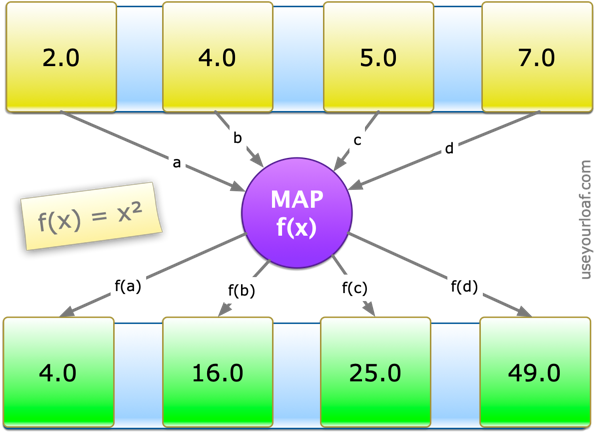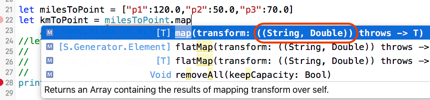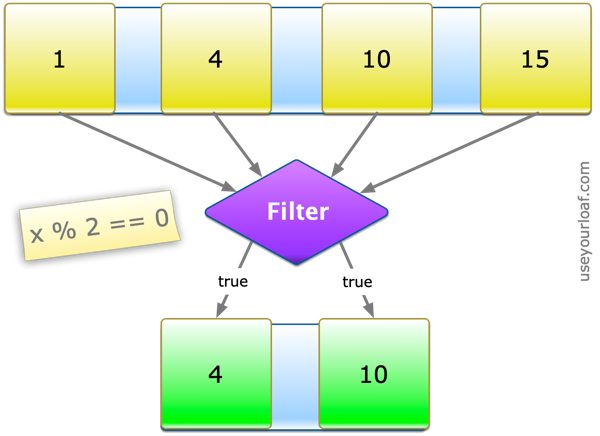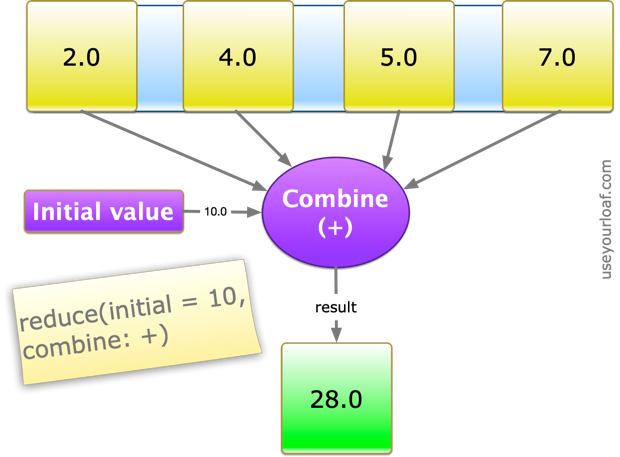## Swift Guide to Map Filter Reduce

Using `map`, `filter` or `reduce` to operate on Swift collection types such as `Array` or `Dictionary` is something that can take getting used to. Unless you have experience with functional languages your instinct may be to reach for the more familiar for-in loop. With that in mind here is my guide to using `map`, `filter`, `reduce` (and `flatMap` and `compactMap`).

Last updated: May 1, 2018

### Map

Use `map` to loop over a collection and apply the same operation to each element in the collection. The `map` function returns an array containing the results of applying a mapping or transform function to each item:We could use a for-in loop to compute the squares of each item in an array:

``````let values = [2.0,4.0,5.0,7.0]
var squares: [Double] = []
for value in values {
squares.append(value*value)
}
``````

This works but the boilerplate code to declare the type of the `squares` array and then loop over it is a little verbose. We also need to make the `squares` array a var as we are changing it in the loop. Now compare to when we use `map`:

``````let squares2 = values.map {\$0 * \$0}
// [4.0, 16.0, 25.0, 49.0]
``````

This is a big improvement. We don’t need the for loop as `map` takes care of that for us. Also the `squares` result is now a let or non-mutating value and we did not even need to declare its type as Swift can infer it.

The shorthand closure syntax can make this hard to follow at first. The `map` function has a single argument which is a closure (a function) that it calls as it loops over the collection. This closure takes the element from the collection as an argument and returns a result. The map function returns these results in an array.

Writing the mapping function in long form can make it easier to see what is happening:

``````let squares3 = values.map({
(value: Double) -> Double in
return value * value
})
``````

The closure has a single argument: `(value: Double)` and returns a `Double` but Swift can infer this. Also since `map` has a single argument which is a closure we do not need the `(` and `)` and with a single line closure we can even omit the `return`:

``````let squares4 = values.map {value in value * value}
``````

The `in` keyword separates the argument from the body of the closure. If you prefer you can go one step further and use the numbered arguments shorthand:

``````let squares5 = values.map { \$0 * \$0 }
``````

The type of the results is not limited to the type of the elements in the original array. Here is an example of mapping an array of integers to strings:

``````let scores = [0,28,124]
let words = scores.map { NumberFormatter.localizedString(from: \$0 as NSNumber, number: .spellOut) }
// ["zero", "twenty-eight", "one hundred twenty-four"]
``````

The map operation is not limited to Arrays you can use it anywhere you have a collection type. For example, use it with a `Dictionary` or a `Set`, the result will always be an `Array`. Here is an example with a Dictionary:

``````let milesToPoint = ["point1":120.0,"point2":50.0,"point3":70.0]
let kmToPoint = milesToPoint.map { name,miles in miles * 1.6093 }
``````

Quick tip: If you have trouble understanding the argument types of the closure Xcode code completion will help you:In this case we are mapping a `Dictionary` so as we iterate over the collection our closure has arguments that are a `String` and a `Double` from the types of the key and value that make up each element of the dictionary.

### Filter

Use `filter` to loop over a collection and return an `Array` containing only those elements that match an include condition.The `filter` method has a single argument that specifies the include condition. This is a closure that takes as an argument the element from the collection and must return a `Bool` indicating if the item should be included in the result.

An example that filters as array of integers returning only the even values:

``````let digits = [1,4,10,15]
let even = digits.filter { \$0 % 2 == 0 }
// [4, 10]
``````

### Reduce

Use `reduce` to combine all items in a collection to create a single new value.The `reduce` method takes two values, an initial value and a combine closure. For example, to add the values of an array to an initial value of 10.0:

``````let items = [2.0,4.0,5.0,7.0]
let total = items.reduce(10.0, +)
// 28.0
``````

This will also work with strings using the `+` operator to concatenate:

``````let codes = ["abc","def","ghi"]
let text = codes.reduce("", +)
// "abcdefghi"
``````

The combine argument is a closure so you can also write reduce using the trailing closure syntax:

``````let names = ["alan","brian","charlie"]
let csv = names.reduce("===") {text, name in "\(text),\(name)"}
// "===,alan,brian,charlie"
``````

### FlatMap and CompactMap

These are variations on the plain `map` that flatten or compact the result. There are three situations where they apply:

1. Using `FlatMap` on a sequence with a closure that returns a sequence:

``````Sequence.flatMap<S>(_ transform: (Element) -> S)
-> [S.Element] where S : Sequence
``````

I think this was probably the first use of `flatMap` I came across in Swift. Use it to apply a closure to each element of a sequence and flatten the result:

``````let results = [[5,2,7], [4,8], [9,1,3]]
let allResults = results.flatMap { \$0 }
// [5, 2, 7, 4, 8, 9, 1, 3]

let passMarks = results.flatMap { \$0.filter { \$0 > 5} }
// [7, 8, 9]
``````

2. Using `FlatMap` on an optional:

The closure takes the non-nil value of the optional and returns an optional.

``````Optional.flatMap<U>(_ transform: (Wrapped) -> U?) -> U?
``````

If the original optional is `nil` then `flatMap` returns `nil`:

``````let input: Int? = Int("8")
let passMark: Int? = input.flatMap { \$0 > 5 ? \$0 : nil }
// 8
``````

3. Using `CompactMap` on a sequence with a closure that returns an optional:

``````Sequence.compactMap<U>(_ transform: (Element) -> U?) -> U?
``````

Note that this use of `flatMap` was renamed to `compactMap` in Swift 4.1 (Xcode 9.3). It provides a convenient way to strip `nil` values from an array:

``````let keys: [String?] = ["Tom", nil, "Peter", nil, "Harry"]
let validNames = keys.compactMap { \$0 }
validNames
// ["Tom", "Peter", "Harry"]

let counts = keys.compactMap { \$0?.count }
counts
// [3, 5, 5]
``````

### Chaining

You can chain methods. For example to sum only those numbers greater than or equal to seven we can first filter and then reduce:

``````let marks = [4,5,8,2,9,7]
let totalPass = marks.filter{\$0 >= 7}.reduce(0, +)
// 24
``````

Another example that returns only the even squares by first filtering the odd values and then mapping the remaining values to their squares (as pointed out in the comments filtering first avoids mapping the odd values which always give odd squares):

``````let numbers = [20,17,35,4,12]
let evenSquares = numbers.filter{\$0 % 2 == 0}.map{\$0 * \$0}
// [400, 16, 144]
``````

### Quick Summary

Next time you find yourself looping over a collection check if you could use map, filter or reduce:

• `map` returns an `Array` containing results of applying a transform to each item.
• `filter` returns an `Array` containing only those items that match an include condition.
• `reduce` returns a single value calculated by calling a combine closure for each item with an initial value.

### Xcode Playground

This post is also available as an Xcode playground in my GitHub repository: